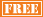January 26
March 9
May 4
June 1

February 9
April 13
June 8

April 20

• ## 2013 GED Test Dates

Varies by State

• ## 2013 AP Exams Test Dates

Calculus AB May 8
Calculus BC May 8
Statistics May 10
Macroeconomics May 16
Microeconomics May 16

# GMAT Math

We provide intensive tutoring for students preparing for the mathematics section of the GMAT exam. The math section consists of 37 questions including problem solving and data sufficiency type questions to be completed in 75 minutes. The problems test students on basic arithmetic, algebra, and geometry although the questions can be quite difficult. The scoring on the math section ranges from 0-60 and the total composite score on the GMAT Exam ranges from 200 to 800.

We utilize a three phase attack to help students properly prepare for the math section of the GMAT Exam. During the course of tutoring sessions, we review numerous simulated GMAT type problems covering the math topics for which students are responsible. We teach problem solving strategies and techniques which students can apply to specific types of questions on the exam. And we reinforce GMAT syllabus math topics which arise during the review of simulated problems for which students are not sufficiently knowledgeable. These 3 components of our GMAT prep program provide students with exam problem recognition the day of the exam, and the necessary underlying math foundation and strategies to solve problems efficiently and correctly.

We offer a dynamic, intensive program for students preparing for the math section of the GMAT Exam. Our focus and expertise is the math section of the exam. All of our efforts go into the single goal of helping students achieve their highest possible score on the math section of the GMAT Exam.

• ## One-on-One GMAT Math Program includes:

Initial student consultationInitial student assessmentNumber of sessions determined by student

Review of simulated GMAT math problems

Review of strategies for attacking GMAT math problems

Review of GMAT math syllabus

Homework review following each session

• ## Group GMAT Math Program includes:

Initial student consultationInitial student assessmentClass size of no more than 20 students

Review of simulated GMAT math problems

Review of strategies for attacking GMAT math problems

Review of GMAT math syllabus